# Nickzom Calculator Calculates the Centroid or Centre of Gravity of a Semicircle | Statics (Mechanics)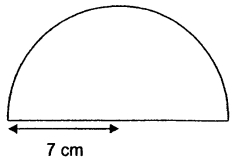The image above is a semicircle with a radius of 7 cm.
To compute the centroid or centre of gravity of a semicircle, you need one essential parameter and this parameter is the radius of the semicircle.

The formula for calculating the centroid or centre of gravity of a semicircle is:

C.G.= 4r /

Where:
r = Radius of the Semicircle
π = Mathematical Constant = 3.142 (approximately)

Let’s solve an example:
Find the centroid or centre of gravity of a semicircle where the radius is 7 cm.

From the example above,
r = radius of the semicircle = 7

C.G. = 4(7) /
C.G. = 28 / 9.4247
C.G. = 2.97

Therefore, the centroid or centre of gravity of the semicircle is 2.97.

Nickzom Calculator – The Calculator Encyclopedia is capable of calculating the centre of gravity of a semicircle at a distance from its base measured along the vertical radius.

To get the answer and workings of the center of gravity or centroid of a semicircle using the Nickzom Calculator – The Calculator Encyclopedia. First, you need to obtain the app.

You can get this app via any of these means:

To get access to the professional version via web, you need to register and subscribe for NGN 1,500 per annum to have utter access to all functionalities.
You can also try the demo version via https://www.nickzom.org/calculator

Once, you have obtained the calculator encyclopedia app, proceed to the Calculator Map, then click on Mechanics under the Engineering sectionNow, click on Centre of Gravity under StaticsNow, click on Centre of Gravity of a Semicircle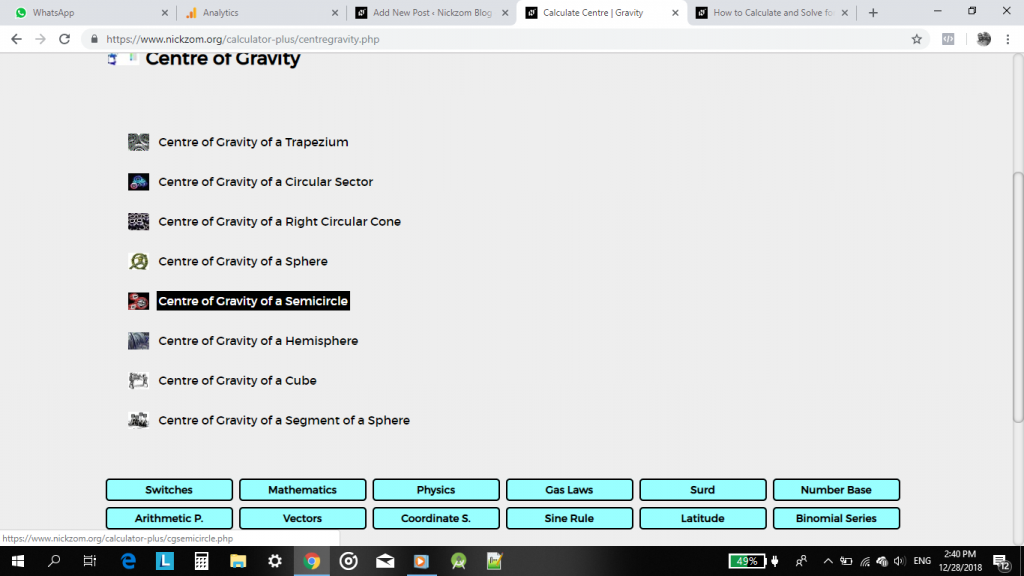The screenshot below displays the page or activity to enter your value, to get the answer for the centre of gravity of a semicircle according to the respective parameter which is the radius of the semicircle (r).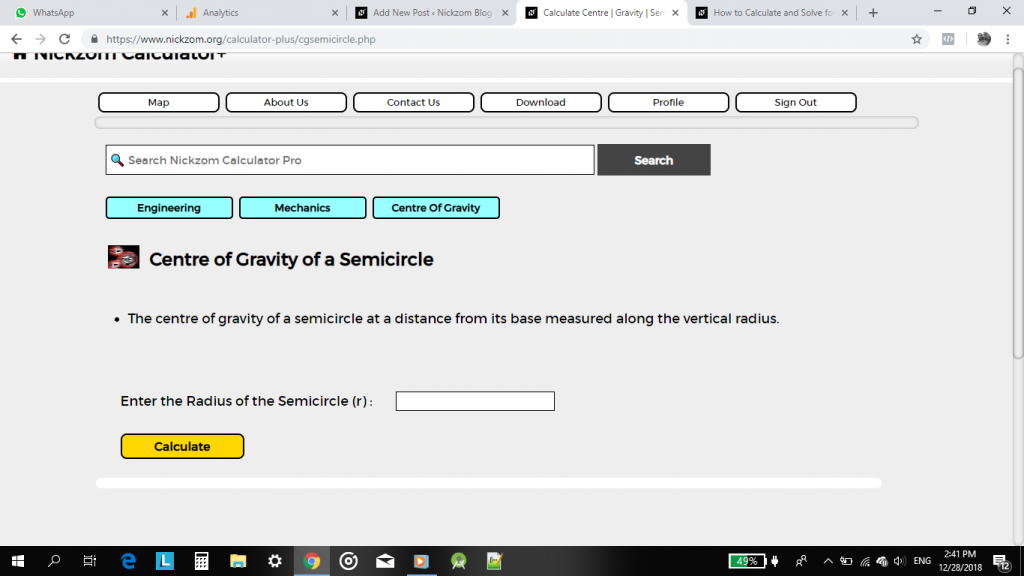Now, enter the value appropriately and accordingly for the parameter as required by the example above where the radius of the semicircle is 7.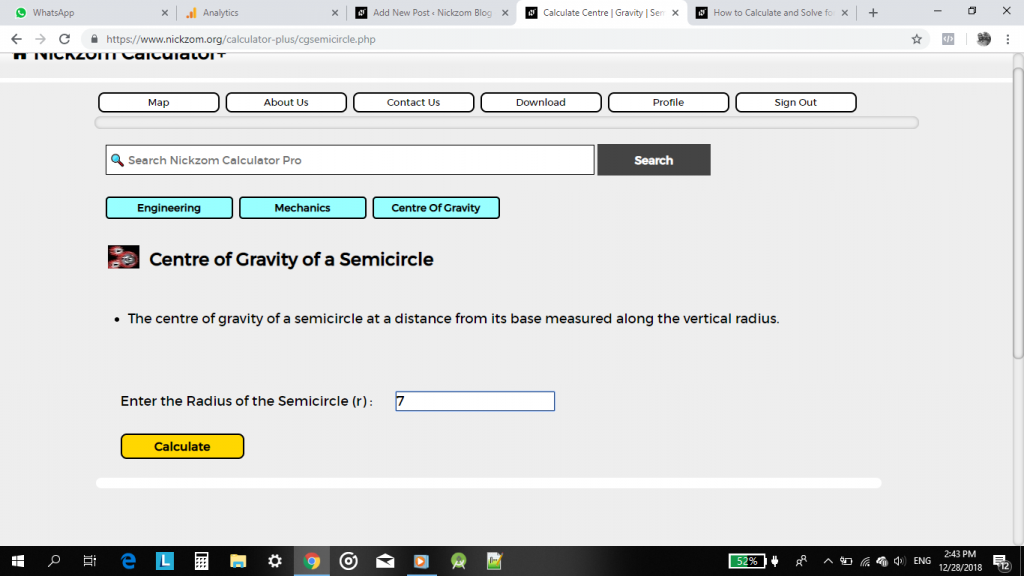Finally, click on Calculate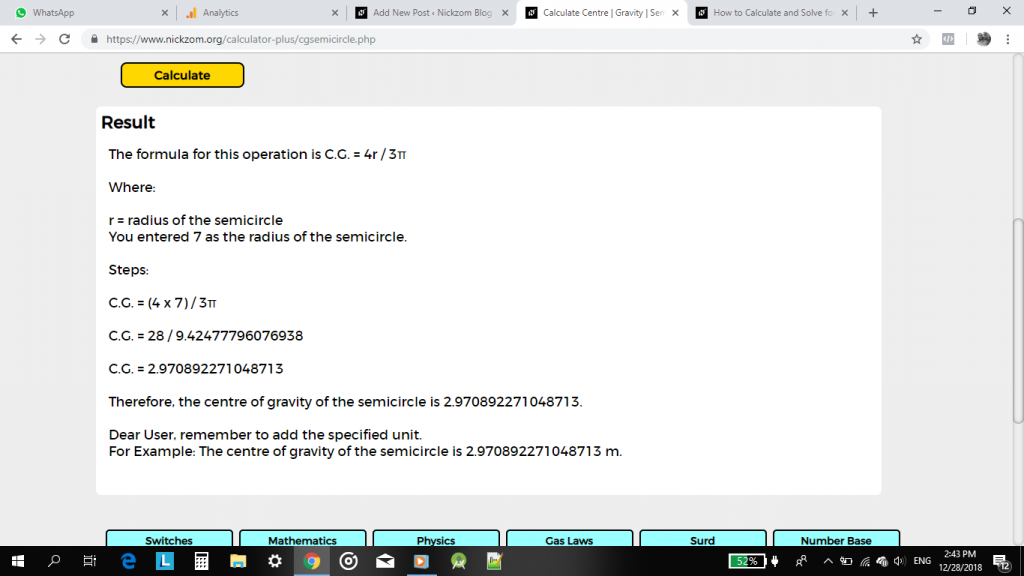As you can see from the screenshot above, Nickzom Calculator – The Calculator Encyclopedia solves for the centre of gravity or centroid of a semicircle and presents the formula, workings and steps too.